# 3-02 TensorFlow入门-训练分类器# 训练逻辑分类器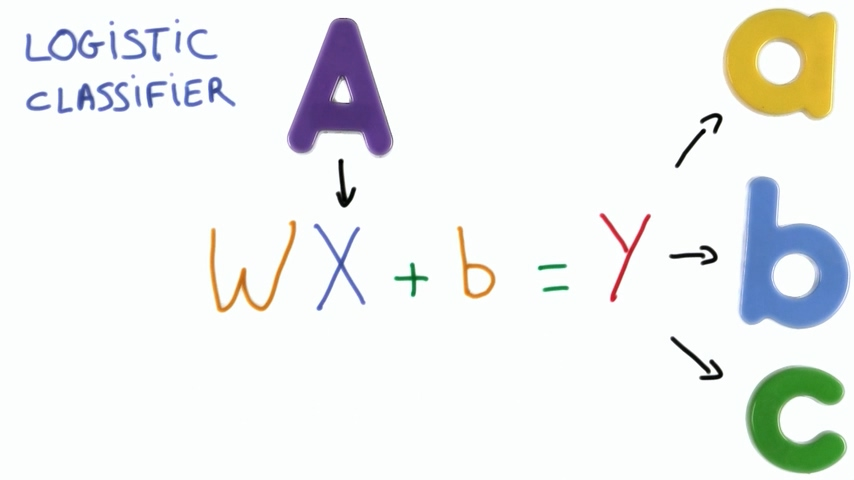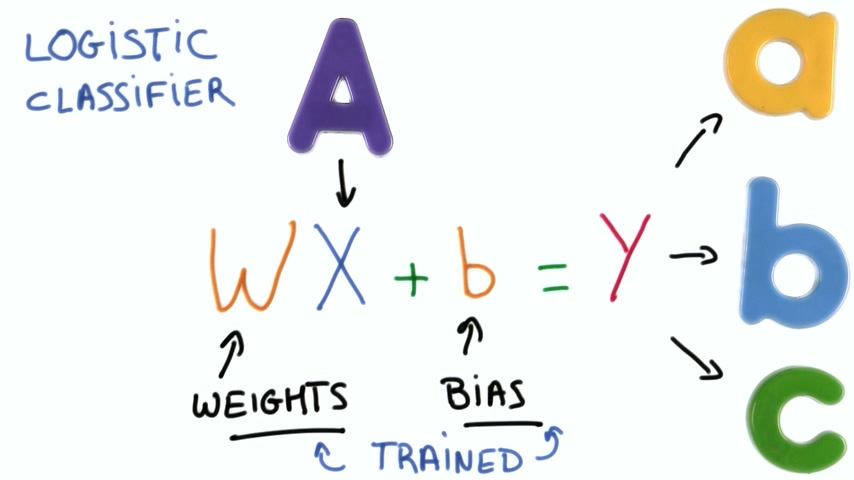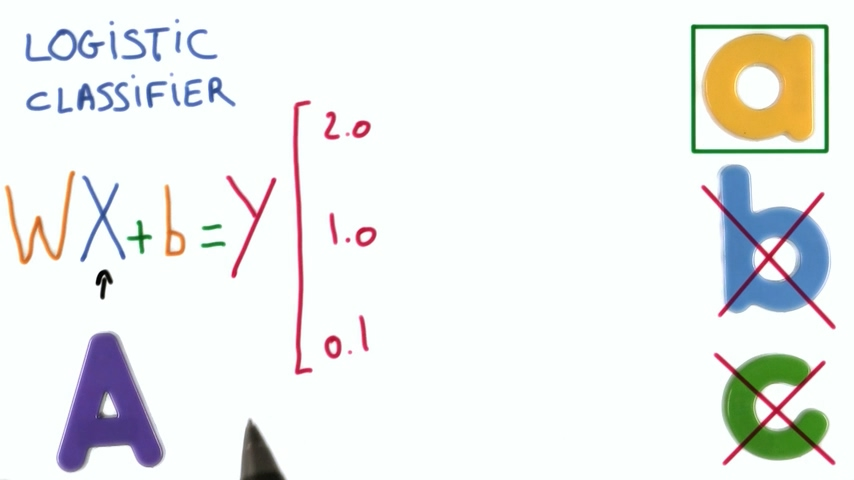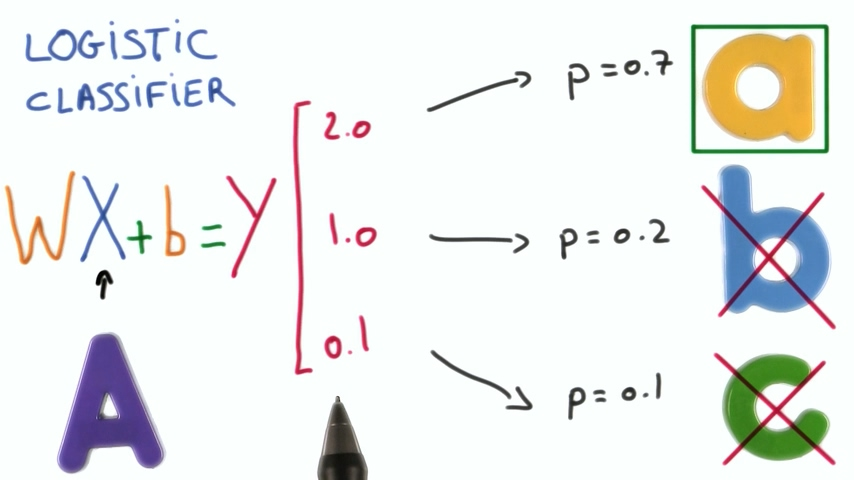## Softmax方法

Softmax函数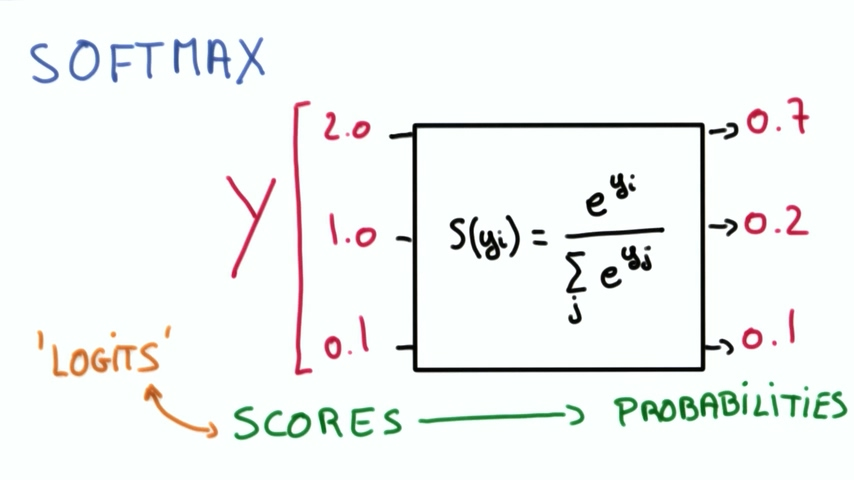# TensorFlow的线性方程

X是我们的像素点列表，y是归类，每一个归类代表一个数字。首先看看y=wX，也就是权重如何影响x对y的预测。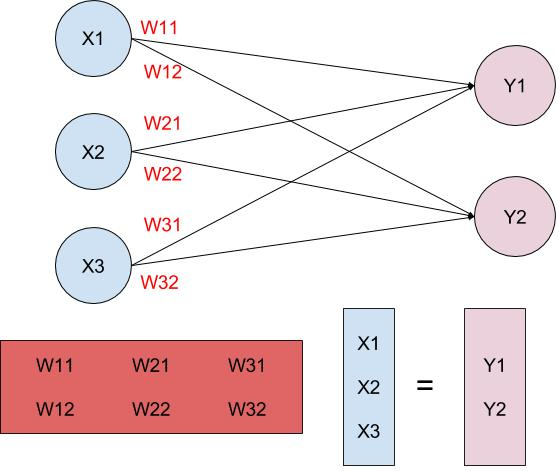y=wX已经可以让我们把数据分类到不同的标签内了，然而这个公式还有个问题就是当x输入为0的时候y一定输出0，这与实际不符。我们希望能够避开这个问题，因此我们引入了偏置b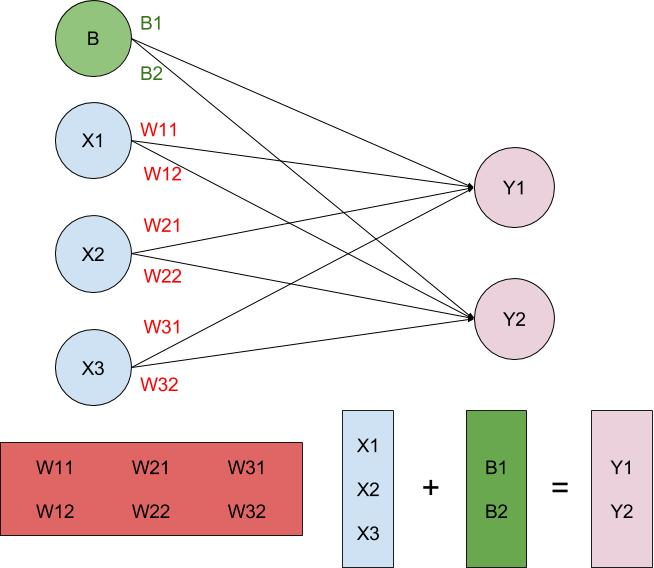y=wX+b允许我们去创建预测了。

## 矩阵的转置

y=wX+b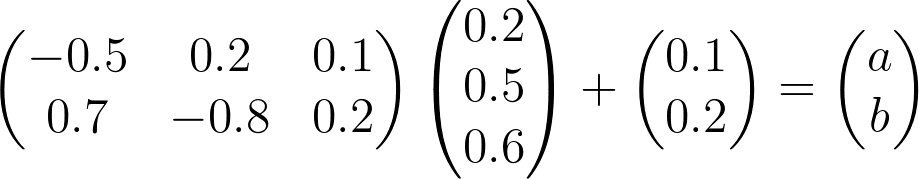y=Xw+b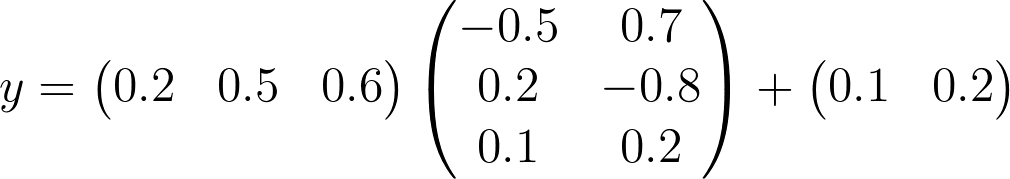# TensorFlow中的权重和偏置

``````x = tf.Variable(5)
``````

tf.Variable创建一个带有初始值的Tensor，就和我们Python里的变量很像。所有的变量都必须进行初始化，我们可以用tf.global_variables_initializer()对所有变量进行初始化。

``````init = tf.global_variables_initializer()
with tf.Session() as sess:
sess.run(init)
``````

``````n_features = 120
n_labels = 5
weights = tf.Variable(tf.truncated_normal((n_features, n_labels)))
``````

tf.truncated_normal函数返回一个Tensor，这个Tensor具有正太分布的随机值，它的幅度和平均值的偏差不超过2个标准差。由于权重已经被随机初始化了，我们不用担心每次训练都从同一个地方开始，因此偏置值不需要随机初始化，可以全部设置初始值为0.

``````n_labels = 5
bias = tf.Variable(tf.zeros(n_labels))
``````

# 实战：识别手写0,1,2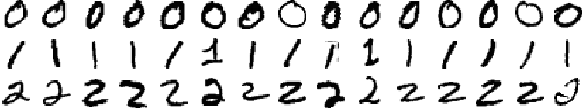## 代码

``````# Solution is available in the other "quiz_solution.py" tab
import tensorflow as tf

def get_weights(n_features, n_labels):
"""
Return TensorFlow weights
:param n_features: Number of features
:param n_labels: Number of labels
:return: TensorFlow weights
"""
# TODO: Return weights
weights = tf.Variable(tf.truncated_normal((n_features, n_labels)))
return weights
pass

def get_biases(n_labels):
"""
Return TensorFlow bias
:param n_labels: Number of labels
:return: TensorFlow bias
"""
# TODO: Return biases
bias = tf.Variable(tf.zeros(n_labels))
return bias
pass

def linear(input, w, b):
"""
Return linear function in TensorFlow
:param input: TensorFlow input
:param w: TensorFlow weights
:param b: TensorFlow biases
:return: TensorFlow linear function
"""
# TODO: Linear Function (xW + b)
return logits
pass
``````

Github链接